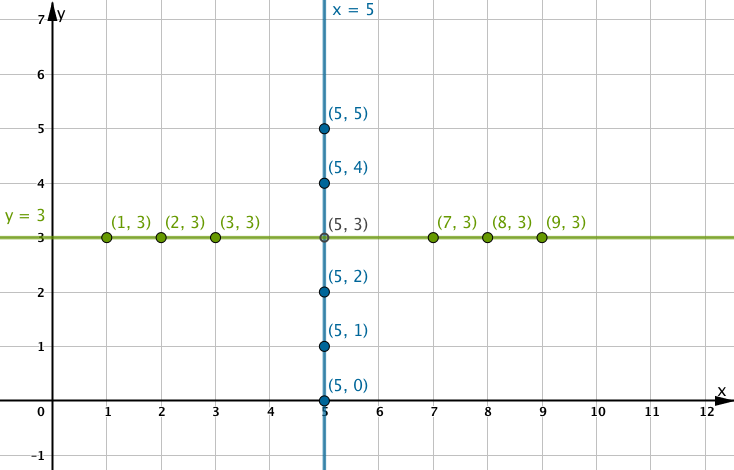# Vertical and Horizontal Lines

The vertical lineis drawn in blue. Notice that the x coordinate of all the points on the blue line is 5.

The horizontal lineis drawn in green. Notice that the y coordinate of all the points on the green line is 3.# Parallel Lines

Parallel lines have the same slope.

Move the slider ‘b’ to see a set of lines that all have the same slope.

# Perpendicular Lines

Suppose we sit a box that measures 5 by 3 on the x axis as shown below. The diagonal line drawn has slope -5/3.

Rotate the box to see that when a line is rotated a full 90 degrees, the rise and run are interchanged, and a negative slope becomes a positive slope.

In general, if slope of line 1 isand if slope of line 2 isthen lines 1 and 2 are perpendicular to each other.

# Try it!

Use any method to figure out the equation of the green line so that it goes through the point marked P and is perpendicular to the blue line.